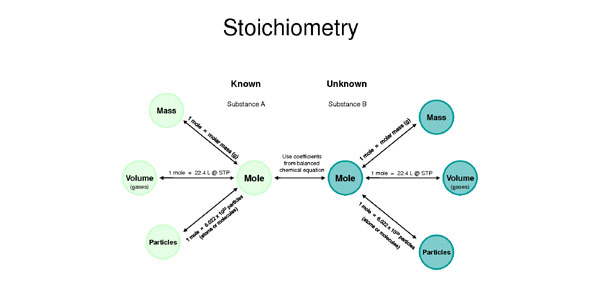# Topic 7: Stoichiometry Part 1

10 Questions | Total Attempts: 274Settings• 1.
How many molecules of water are there in 36.0 grams of the substance?
• A.

6.0 X 10^23

• B.

1.2 X 10^24

• C.

2.8X 10^23

• D.

3.2 X 10^21

• E.

1.8 X 10^24

• 2.
Molar Mass of glucose (C6H12O6) in grams per mole?
• A.

340.0

• B.

180.0

• C.

110.0

• D.

78.0

• E.

44.0

• 3.
Molar Mass of glucose (CO2) in grams per mole?
• A.

340.0

• B.

180.0

• C.

110.0

• D.

78.0

• E.

44.0

• 4.
What is the molar mass of H2  i in grams per mole
• A.

15.0

• B.

2.0

• C.

4.0

• D.

5.2

• E.

3.5

• 5.
What is the molar mass of NOin grams per mole
• A.

16.2

• B.

46.0

• C.

44.0

• D.

13.1

• E.

None of the above

• 6.
What is the molar mass of benzene C6H6 ?( in grams per mole)
• A.

44.0

• B.

33.1

• C.

57.2

• D.

46.9

• E.

78.0

• 7.
1.8 X 10^24 atoms of  sodium contains approximately how many grams of this substance?
• A.

83

• B.

69

• C.

17

• D.

11

• E.

23

• 8.
6.02 X 10^23 is known as
• A.

Charles' number

• B.

Irrational number

• C.

• D.

Boyle's law

• E.

None of the above

• 9.
What is the molar mass of NH3? ( in grams per mole)
• A.

13

• B.

14

• C.

15

• D.

17

• E.

20

• 10.
What is the molar mass of CH4
• A.

16 grams per mole

• B.

20 grams per mole

• C.

24 grams per mole

• D.

30 grams per mole

• E.

14 grams per mole

Related TopicsBack to top